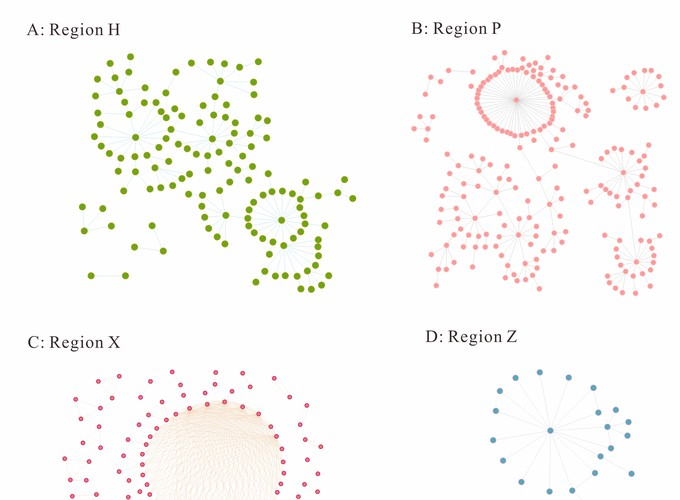Source

# A comparative study on the three calculation methods for reproduction numbers of COVID-19

### 摘要

Objective: This study uses four COVID-19 outbreaks as examples to calculate and compare merits and demerits, as well as applicational scenarios, of three methods for calculating reproduction numbers. Method: The epidemiological characteristics of the COVID-19 outbreaks are described. Through the definition method, the next-generation matrix-based method, and the epidemic curve and serial interval (SI)-based method, corresponding reproduction numbers were obtained and compared. Results: Reproduction numbers (Reff), obtained by the definition method of the four regions, are 1.20, 1.14, 1.66, and 1.12. Through the next generation matrix method, in region H Reff = 4.30, 0.44; region P Reff = 6.5, 1.39, 0; region X Reff = 6.82, 1.39, 0; and region Z Reff = 2.99, 0.65. Time-varying reproduction numbers (Rt), which are attained by SI of onset dates, are decreasing with time. Region H reached its highest Rt = 2.8 on July 29 and decreased to Rt < 1 after August 4; region P reached its highest Rt = 5.8 on September 9 and dropped to Rt < 1 by September 14; region X had a fluctuation in the Rt and Rt < 1 after September 22; Rt in region Z reached a maximum of 1.8 on September 15 and decreased continuously to Rt < 1 on September 19. Conclusion: The reproduction number obtained by the definition method is optimal in the early stage of epidemics with a small number of cases that have clear transmission chains to predict the trend of epidemics accurately. The effective reproduction number Reff, calculated by the next generation matrix, could assess the scale of the epidemic and be used to evaluate the effectiveness of prevention and control measures used in epidemics with a large number of cases. Time-varying reproduction number Rt, obtained via epidemic curve and SI, can give a clear picture of the change in transmissibility over time, but the conditions of use are more rigorous, requiring a greater sample size and clear transmission chains to perform the calculation. The rational use of the three methods for reproduction numbers plays a role in the further study of the transmissibility of COVID-19.

Frontiers in Medicine